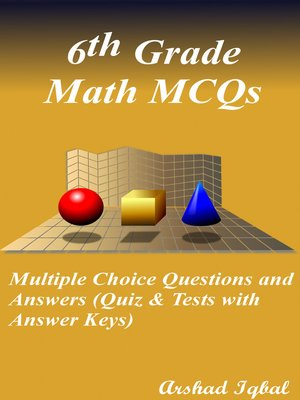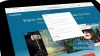### Multiple Choice Questions and Answers (Quiz & Tests with Answer Keys)

ebook

With an OverDrive account, you can save your favorite libraries for at-a-glance information about availability. Find out more about OverDrive accounts.

### Subjects

6th grade math multiple choice questions has 448 MCQs. Grade 6 math quiz questions and answers pdf, MCQs on integers, rational numbers, sequence and series, factors and multiples, volume and surface area, functions, graphs, angle properties of polygons, class 6 mathematics MCQs with answers, estimation and approximation, fundamental algebra, algebraic equations and simple inequalities, arithmetical problems and percentages, ratio rate and speed, geometrical concepts and properties, perimeter and area of geometrical figures MCQs and quiz worksheets to practice exam prep tests.
6th grade math multiple choice quiz questions and answers pdf, math exam revision and study guide with practice tests for online exam prep and interviews. Math interview questions and answers to ask, to prepare and to study for jobs interviews and career MCQs with answer keys.
Algebraic equations and simple inequalities quiz has 69 multiple choice questions. Angle properties of polygons quiz has 17 multiple choice questions. Arithmetical problems and percentages quiz has 48 multiple choice questions with answers. Estimation and approximation quiz has 31 multiple choice questions. Factors and multiples quiz has 41 multiple choice questions. Functions and graphs quiz has 17 multiple choice questions.
Fundamental algebra quiz has 70 multiple choice questions. Geometrical concepts and properties quiz has 24 multiple choice questions. Integer's multiple choice questions for fresh graduates has 42 multiple choice questions. Number sequences quiz has 12 multiple choice questions. Perimeter and area of geometrical figures quiz has 20 multiple choice questions. Ratio rate and speed quiz has 46 multiple choice questions. Rational numbers quiz has 32 multiple choice questions. Volume and surface area quiz has 19 multiple choice questions and answers.
Math interview questions and answers pdf, MCQs on tax calculations, polygons, time calculation, least common multiple, rational numbers, cylinders, complementary angles, prime factorization, significant figures, supplementary angles, math formulas, number line, adjacent angles, algebraic expressions, ratio calculations, discount calculations, types of triangles, Cartesian plane, rounding numbers, average speed, highest common factor, how to do percentages, prime and composite numbers, types of angles, convex polygons, number sequences, addition and subtraction, finding coordinates, algebra rules, factors and multiples, rounding off numbers, commission calculations, index notation, ratio examples, addition of integers, equations and inequalities, percentage of number, rules of integers, subtraction of integers, units of area, algebraic notation, examples of equations, writing algebraic expressions, average rate, geometric concepts, multiplication of integers, squares and square roots, division of integers, solving simple equations, cubes and cube roots, volume of fluids, making formula, rate calculations, absolute value of integer, evaluation of algebraic expressions, factorization by grouping, percentage comparison, distributive law of multiplication, estimation and rounding, multiplication and division of rational numbers, line rays and segments, terminating and recurring decimals, percentage fractions and decimals, ordering of rational numbers, problem solving with algebra, arithmetical operations on rational numbers, brackets in simplification, class 6 factorization, expressing quantities and percentage, idea of functions, increasing decreasing quantities, inequalities learning, linear algebraic expressions and fractional coefficients, ratio increase and decrease, real numbers calculations, round off values, simple equations solutions, grade 6...

### Publication Details

Publisher:
Imprint:
Smashwords Edition
Publication Date:
2016

### Format

• Kindle Book